# fevd

Generate vector error-correction (VEC) model forecast error variance decomposition (FEVD)

## Syntax

``Decomposition = fevd(Mdl)``
``Decomposition = fevd(Mdl,Name=Value)``
``[Decomposition,Lower,Upper] = fevd(___)``
``Tbl = fevd(___)``

## Description

The `fevd` function returns the forecast error decomposition (FEVD) of the variables in a VEC(p – 1) model attributable to shocks to each response variable in the system. A fully specified `vecm` model object characterizes the VEC model.

The FEVD provides information about the relative importance of each innovation in affecting the forecast error variance of all response variables in the system. In contrast, the impulse response function (IRF) traces the effects of an innovation shock to one variable on the response of all variables in the system. To estimate the IRF of a VEC model characterized by a `vecm` model object, see `irf`.

You can supply optional data, such as a presample, as a numeric array, table, or timetable. However, all specified input data must be the same data type. When the input model is estimated (returned by `estimate`), supply the same data type as the data used to estimate the model. The data type of the outputs matches the data type of the specified input data.

example

````Decomposition = fevd(Mdl)` returns a numeric array containing the orthogonalized FEVDs of the response variables that compose the VEC(p – 1) model `Mdl` characterized by a fully specified `vecm` model object. `fevd` shocks variables at time 0, and returns the FEVD for times 1 through 20.If `Mdl` is an estimated model (returned by `estimate`) fit to a numeric matrix of input response data, this syntax applies.```

example

````Decomposition = fevd(Mdl,Name=Value)` uses additional options specified by one or more name-value arguments. `fevd` returns numeric arrays when all optional input data are numeric arrays. For example, `fevd(Mdl,NumObs=10,Method="generalized")` specifies estimating a generalized FEVD for periods 1 through 10.If `Mdl` is an estimated model fit to a numeric matrix of input response data, this syntax applies.```

example

````[Decomposition,Lower,Upper] = fevd(___)` returns numeric arrays of lower `Lower` and upper `Upper` 95% confidence bounds for confidence intervals on the true FEVD, for each period and variable in the FEVD, using any input argument combination in the previous syntaxes. By default, `fevd` estimates confidence bounds by conducting Monte Carlo simulation.If `Mdl` is an estimated model fit to a numeric matrix of input response data, this syntax applies.If `Mdl` is a custom `vecm` model object (an object not returned by `estimate` or modified after estimation), `fevd` can require a sample size for the simulation `SampleSize` or presample responses `Y0`.```

example

````Tbl = fevd(___)` returns the table or timetable `Tbl` containing the FEVDs and, optionally, corresponding 95% confidence bounds, of the response variables that compose the VEC(p – 1) model `Mdl`. The FEVD of the corresponding response is a variable in `Tbl` containing a matrix with columns corresponding to the variables in the system shocked at time 0.If you set at least one name-value argument that controls the 95% confidence bounds on the FEVD, `Tbl` also contains a variable for each of the lower and upper bounds. For example, `Tbl` contains confidence bounds when you set the `NumPaths` name-value argument.If `Mdl` is an estimated model fit to a table or timetable of input response data, this syntax applies.```

## Examples

collapse all

Fit a 4-D VEC(2) model with two cointegrating relations to Danish money and income rate series data in a numeric matrix. Then, estimate and plot the orthogonalized FEVD from the estimated model.

Load the Danish money and income data set.

`load Data_JDanish`

For more details on the data set, enter `Description` at the command line.

Create a `vecm` model object that represents a 4-D VEC(2) model with two cointegrating relations. Specify the variable names.

```Mdl = vecm(4,2,2); Mdl.SeriesNames = DataTable.Properties.VariableNames;```

`Mdl` is a `vecm` model object specifying the structure of a 4-D VEC(2) model; it is a template for estimation.

Fit the VEC(2) model to the numeric matrix of time series data `Data`.

`Mdl = estimate(Mdl,Data);`

`EstMdl` is a fully specified `vecm` model object representing an estimated 4-D VEC(2) model.

Estimate the orthogonalized FEVD from the estimated VEC(2) model.

`Decomposition = fevd(Mdl);`

`Decomposition` is a 20-by-4-by-4 array representing the FEVD of `Mdl`. Rows correspond to consecutive time points from time 1 to 20, columns correspond to variables receiving a one-standard-deviation innovation shock at time 0, and pages correspond to variables whose forecast error variance `fevd` decomposes. `Mdl.SeriesNames` specifies the variable order. By default, `fevd` uses the H1 Johansen form, which is the same default form that `estimate` uses.

Because `Decomposition` represents an orthogonalized FEVD, rows should sum to `1`. This characteristic illustrates that orthogonalized FEVDs represent proportions of variance contributions. Confirm that all rows of `Decomposition` sum to `1`.

```rowsums = sum(Decomposition,2); sum((rowsums - 1).^2 > eps)```
```ans = ans(:,:,1) = 0 ans(:,:,2) = 0 ans(:,:,3) = 0 ans(:,:,4) = 0 ```

Row sums among the pages are close to 1.

Display the contributions to the forecast error variance of the bond rate when real income is shocked at time 0.

`Decomposition(:,2,3)`
```ans = 20×1 0.0694 0.1744 0.1981 0.2182 0.2329 0.2434 0.2490 0.2522 0.2541 0.2559 ⋮ ```

The `armafevd` function plots the FEVD of VAR models characterized by AR coefficient matrices. Plot the FEVD of a VEC model by:

1. Expressing the VEC(2) model as a VAR(3) model by passing `Mdl` to `varm`

2. Passing the VAR model AR coefficients and innovations covariance matrix to `armafevd`

Plot the VEC(2) model FEVD for 40 periods.

```VARMdl = varm(Mdl); armafevd(VARMdl.AR,[],InnovCov=VARMdl.Covariance, ... NumObs=40);```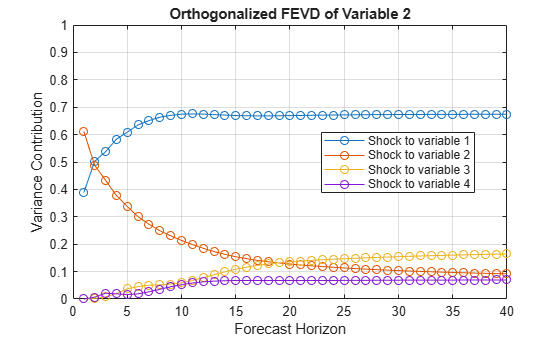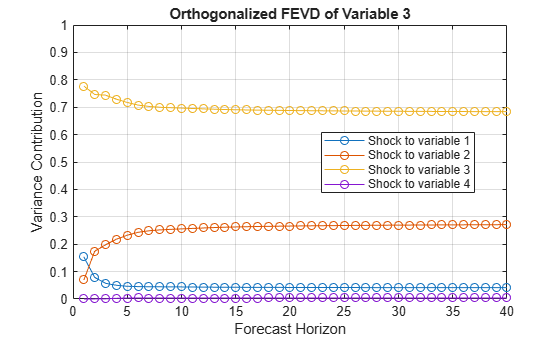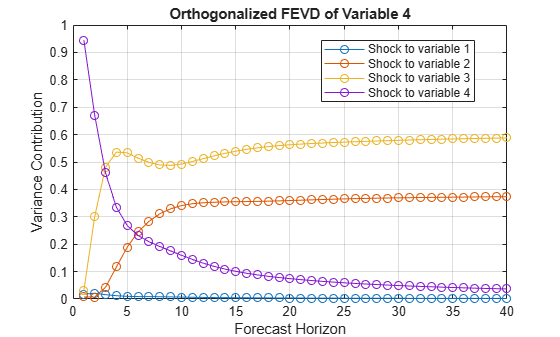Each plot shows the four FEVDs of a variable when all other variables are shocked at time 0. `Mdl.SeriesNames` specifies the variable order.

Consider the 4-D VEC(2) model with two cointegrating relations in Specify Data in Numeric Matrix When Plotting FEVD. Estimate the generalized FEVD of the system for 100 periods.

Load the Danish money and income data set, then estimate the VEC(2) model.

```load Data_JDanish Mdl = vecm(4,2,2); Mdl.SeriesNames = DataTable.Properties.VariableNames; Mdl = estimate(Mdl,DataTable.Series);```

Estimate the generalized FEVD from the estimated VEC(2) model over a forecast horizon with length 100.

`Decomposition = fevd(Mdl,Method="generalized",NumObs=100);`

`Decomposition` is a 100-by-4-by-4 array representing the generalized FEVD of `Mdl`.

Plot the generalized FEVD of the bond rate when real income is shocked at time 0.

```figure; plot(1:100,Decomposition(:,2,3)) title("FEVD of IB When Y Is Shocked") xlabel("Forecast Horizon") ylabel("Variance Contribution") grid on```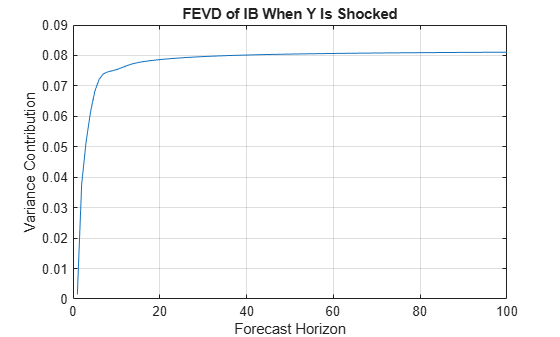When real income is shocked, the contribution of the bond rate to the forecast error variance settles at approximately 0.08.

Fit a 4-D VEC(2) model with two cointegrating relations to Danish money and income rate series data in a timetable. Then, estimate and plot the orthogonalized FEVD and corresponding confidence intervals from the estimated model.

Load the Danish money and income data set.

`load Data_JDanish`

Create a `vecm` model object that represents a 4-D VEC(2) model with two cointegrating relations. Specify the variable names.

```Mdl = vecm(4,2,2); Mdl.SeriesNames = DataTable.Properties.VariableNames;```

`Mdl` is a `vecm` model object specifying the structure of a 4-D VEC(2) model; it is a template for estimation.

Fit the VEC(2) model to the data set.

`EstMdl = estimate(Mdl,DataTimeTable);`

`EstMdl` is a fully specified `vecm` model object representing an estimated 4-D VEC(2) model.

Estimate the orthogonalized FEVD and corresponding 95% confidence intervals from the estimated VEC(2) model. To return confidence intervals, you must set a name-value argument that controls confidence intervals, for example, `Confidence`. Set `Confidence` to `0.95`.

```rng(1); % For reproducibility Tbl = fevd(EstMdl,Confidence=0.95); Tbl.Time(1)```
```ans = datetime 01-Oct-1974 ```
`size(Tbl)`
```ans = 1×2 20 12 ```

`Tbl` is a timetable with 20 rows, representing the periods in the FEVD, and 12 variables. Each variable is a 20-by-4 matrix of the FEVD or confidence bound associated with a variable in the model `EstMdl`. For example, `Tbl.M2_FEVD(:,2)` is the FEVD of `M2` resulting from a 1-standard-deviation shock on `01-Jul-1974` (period 0) to `Mdl.SeriesNames(2)`, which is the variable `Y`. [`Tbl.M2_FEVD_LowerBound(:,2)`,`Tbl.M2_FEVD_UpperBound(:,2)`] are the corresponding 95% confidence intervals. By default, `fevd` uses the H1 Johansen form, which is the same default form that `estimate` uses.

Plot the FEVD of M2 and its 95% confidence interval resulting from a 1-standard-deviation shock on `01-Jul-1974` (period 0) to `Mdl.SeriesNames(2)`, which is the variable `Y`.

```idxM2 = startsWith(Tbl.Properties.VariableNames,"M2"); M2FEVD = Tbl(:,idxM2); shockIdx = 2; figure hold on plot(M2FEVD.Time,M2FEVD.M2_FEVD(:,shockIdx),"-o") plot(M2FEVD.Time,[M2FEVD.M2_FEVD_LowerBound(:,shockIdx) ... M2FEVD.M2_FEVD_UpperBound(:,shockIdx)],"-o",Color="r") legend("FEVD","95% confidence interval") title('M2 FEVD, Shock to Y') hold off```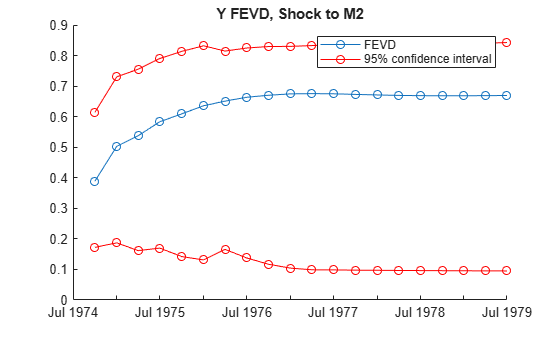Consider the 4-D VEC(2) model with two cointegrating relations in Specify Data in Numeric Matrix When Plotting FEVD. Estimate and plot its orthogonalized FEVD and 95% Monte Carlo confidence intervals on the true FEVD.

Load the Danish money and income data set, then estimate the VEC(2) model.

```load Data_JDanish Mdl = vecm(4,2,2); Mdl.SeriesNames = DataTable.Properties.VariableNames; Mdl = estimate(Mdl,DataTable.Series);```

Estimate the FEVD and corresponding 95% Monte Carlo confidence intervals from the estimated VEC(2) model.

```rng(1); % For reproducibility [Decomposition,Lower,Upper] = fevd(Mdl);```

`Decomposition`, `Lower`, and `Upper` are 20-by-4-by-4 arrays representing the orthogonalized FEVD of `Mdl` and corresponding lower and upper bounds of the confidence intervals. For all arrays, rows correspond to consecutive time points from time 1 to 20, columns correspond to variables receiving a one-standard-deviation innovation shock at time 0, and pages correspond to the variables whose forecast error variance `fevd` decomposes. `Mdl.SeriesNames` specifies the variable order.

Plot the orthogonalized FEVD with its confidence bounds of the bond rate when real income is shocked at time 0.

```fevdshock2resp3 = Decomposition(:,2,3); FEVDCIShock2Resp3 = [Lower(:,2,3) Upper(:,2,3)]; figure; h1 = plot(1:20,fevdshock2resp3); hold on h2 = plot(1:20,FEVDCIShock2Resp3,"r--"); legend([h1 h2(1)],["FEVD" "95% Confidence Interval"],... Location="best") xlabel("Forecast Horizon"); ylabel("Variance Contribution"); title("FEVD of IB When Y Is Shocked"); grid on hold off```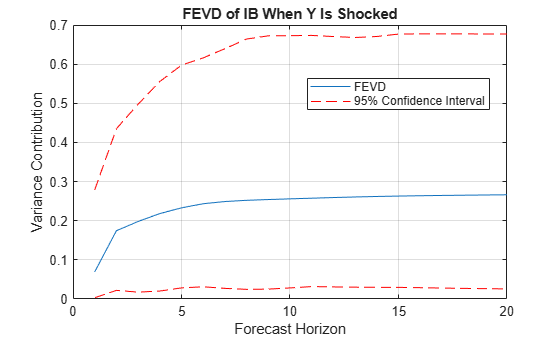In the long run, and when real income is shocked, the proportion of forecast error variance of the bond rate settles between approximately 0 and 0.7 with 95% confidence.

Consider the 4-D VEC(2) model with two cointegrating relations in Specify Data in Numeric Matrix When Plotting FEVD. Estimate and plot its orthogonalized FEVD and 90% bootstrap confidence intervals on the true FEVD.

Load the Danish money and income data set, then estimate the VEC(2) model. Return the residuals from model estimation.

```load Data_JDanish Mdl = vecm(4,2,2); Mdl.SeriesNames = DataTable.Properties.VariableNames; [Mdl,~,~,Res] = estimate(Mdl,DataTable.Series); T = size(DataTable,1) % Total sample size```
```T = 55 ```
`n = size(Res,1) % Effective sample size`
```n = 52 ```

`Res` is a 52-by-4 array of residuals. Columns correspond to the variables in `Mdl.SeriesNames`. The `estimate` function requires `Mdl.P` = 3 observations to initialize a VEC(2) model for estimation. Because presample data (`Y0`) is unspecified, `estimate` takes the first three observations in the specified response data to initialize the model. Therefore, the resulting effective sample size is `T``Mdl.P` = 52, and rows of `Res` correspond to the observation indices 4 through `T`.

Estimate the orthogonalized FEVD and corresponding 90% bootstrap confidence intervals from the estimated VEC(2) model. Draw 500 paths of length `n` from the series of residuals.

```rng(1); % For reproducibility [Decomposition,Lower,Upper] = fevd(Mdl,E=Res,NumPaths=500, ... Confidence=0.9);```

Plot the orthogonalized FEVD with its confidence bounds of the bond rate when real income is shocked at time 0.

```fevdshock2resp3 = Decomposition(:,2,3); FEVDCIShock2Resp3 = [Lower(:,2,3) Upper(:,2,3)]; figure; h1 = plot(0:19,fevdshock2resp3); hold on h2 = plot(0:19,FEVDCIShock2Resp3,'r--'); legend([h1 h2(1)],["FEVD" "90% Confidence Interval"], ... Location="best") xlabel("Time Index"); ylabel("Response"); title("FEVD of IB When Y Is Shocked"); grid on hold off```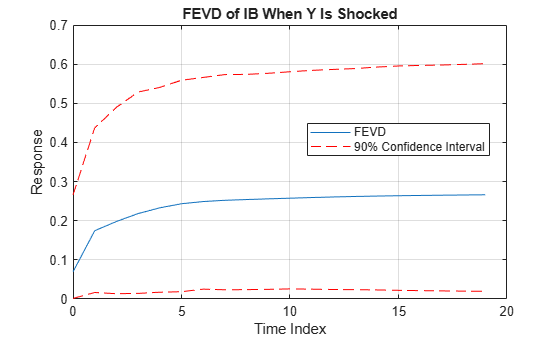In the long run, and when real income is shocked, the proportion of forecast error variance of the bond rate settles between approximately 0 and 0.6 with 90% confidence.

## Input Arguments

collapse all

VEC model, specified as a `vecm` model object created by `vecm` or `estimate`. `Mdl` must be fully specified.

If `Mdl` is an estimated model (returned by `estimate`) , you must supply any optional data using the same data type as the input response data, to which the model is fit.

If `Mdl` is a custom `vecm` model object (an object not returned by `estimate` or modified after estimation), `fevd` can require a sample size for the simulation `SampleSize` or presample responses `Y0`.

### Name-Value Arguments

Specify optional pairs of arguments as `Name1=Value1,...,NameN=ValueN`, where `Name` is the argument name and `Value` is the corresponding value. Name-value arguments must appear after other arguments, but the order of the pairs does not matter.

Before R2021a, use commas to separate each name and value, and enclose `Name` in quotes.

Example: `fevd(Mdl,NumObs=10,Method="generalized",E=Res)` specifies estimating a generalized FEVD for periods 1 through 10, and bootstraps the residuals in the numeric array `Res` to compute the 95% confidence bounds.

Options for All FEVDs

collapse all

Number of periods for which `fevd` computes the FEVD (the forecast horizon), specified as a positive integer. `NumObs` specifies the number of observations to include in the FEVD (the number of rows in `Decomposition`).

Example: `NumObs=10` specifies estimation of the FEVD for times 1 through 10.

Data Types: `double`

FEVD computation method, specified as a value in this table.

ValueDescription
`"orthogonalized"`Compute variance decompositions using orthogonalized, one-standard-deviation innovation shocks. `fevd` uses the Cholesky factorization of `Mdl.Covariance` for orthogonalization.
`"generalized"`Compute variance decompositions using one-standard-deviation innovation shocks.

Example: `Method="generalized"`

Data Types: `char` | `string`

Johansen form of the VEC(p – 1) model deterministic terms , specified as a value in this table (for variable definitions, see Vector Error-Correction Model).

ValueError-Correction TermDescription
`"H2"`

AB´yt − 1

No intercepts or trends are present in the cointegrating relations, and no deterministic trends are present in the levels of the data.

Specify this model only when all response series have a mean of zero.

`"H1*"`

A(B´yt−1+c0)

Intercepts are present in the cointegrating relations, and no deterministic trends are present in the levels of the data.

`"H1"`

A(B´yt−1+c0)+c1

Intercepts are present in the cointegrating relations, and deterministic linear trends are present in the levels of the data.

`"H*"`A(B´yt−1+c0+d0t)+c1

Intercepts and linear trends are present in the cointegrating relations, and deterministic linear trends are present in the levels of the data.

`"H"`A(B´yt−1+c0+d0t)+c1+d1t

Intercepts and linear trends are present in the cointegrating relations, and deterministic quadratic trends are present in the levels of the data.

If quadratic trends are not present in the data, this model can produce good in-sample fits but poor out-of-sample forecasts.

For more details on the Johansen forms, see `estimate`.

Tip

A best practice is to maintain model consistency during the simulation that estimates confidence bounds. Therefore, if `Mdl` is an estimated `vecm` model object (an object returned by `estimate` and unmodified thereafter), incorporate any constraints imposed during estimation by deferring to the default value of `Model`.

Example: `Model="H1*"`

Data Types: `string` | `char`

Options for Confidence Bound Estimation

collapse all

Number of sample paths (trials) to generate, specified as a positive integer.

Example: `NumPaths=1000` generates `1000` sample paths from which the software derives the confidence bounds.

Data Types: `double`

Number of observations for the Monte Carlo simulation or bootstrap per sample path, specified as a positive integer.

• If `Mdl` is an estimated `vecm` model object (an object returned by `estimate` and unmodified thereafter), the default is the sample size of the data to which the model is fit (see `summarize`).

• Otherwise:

• If `fevd` estimates confidence bounds by conducting a Monte Carlo simulation, you must specify `SampleSize`.

• If `fevd` estimates confidence bounds by bootstrapping residuals, the default is the length of the specified series of residuals (`size(Res,1)`, where `Res` is the number of residuals in `E` or `InSample`).

Example: If you specify `SampleSize=100` and do not specify the `E` name-value argument, the software estimates confidence bounds from `NumPaths` random paths of length `100` from `Mdl`.

Example: If you specify `SampleSize=100,E=Res`, the software resamples, with replacement, `100` observations (rows) from `Res` to form a sample path of innovations to filter through `Mdl`. The software forms `NumPaths` random sample paths from which it derives confidence bounds.

Data Types: `double`

Presample response data that provides initial values for model estimation during the simulation, specified as a `numpreobs`-by-`numseries` numeric matrix. Use `Y0` only in the following situations:

`numpreobs` is the number of presample observations. `numseries` is `Mdl.NumSeries`, the dimensionality of the input model.

Each row is a presample observation, and measurements in each row occur simultaneously. The last row contains the latest presample observation. `numpreobs` is the number of specified presample responses and it must be at least `Mdl.P`. If you supply more rows than necessary, `fevd` uses the latest `Mdl.P` observations only.

`numseries` is the dimensionality of the input VEC model `Mdl.NumSeries`. Columns must correspond to the response variables in `Mdl.SeriesNames`.

The following situations determine the default or whether presample response data is required.

• If `Mdl` is an unmodified estimated model, `fevd` sets `Y0` to the presample response data used for estimation by default (see the `Y0` name-value argument of `estimate`).

• If `Mdl` is a custom model and you return confidence bounds `Lower` or `Upper`, you must specify `Y0`.

Data Types: `double`

Presample data that provide initial values for the model `Mdl`, specified as a table or timetable with `numprevars` variables and `numpreobs` rows. Use `Presample` only in the following situations:

• You supply other optional data inputs as tables or timetables.

• `Mdl` is an estimated `vecm` model object (an object returned by `estimate` and unmodified thereafter) fit to a numeric matrix of response data.

Each row is a presample observation, and measurements in each row occur simultaneously. The last row contains the latest presample observation. `numpreobs` is the number of specified presample responses and it must be at least `Mdl.P`. If you supply more rows than necessary, `fevd` uses the latest `Mdl.P` observations only.

Each variable is a `numpreobs` numeric vector representing one path. To control presample variable selection, see the optional `PresampleResponseVariables` name-value argument.

If `Presample` is a timetable, all the following conditions must be true:

• `Presample` must represent a sample with a regular datetime time step (see `isregular`).

• The datetime vector of sample timestamps `Presample.Time` must be ascending or descending.

If `Presample` is a table, the following conditions hold:

• The last row contains the latest presample observation.

• `Presample.Properties.RowsNames` must be empty.

The following situations determine the default or whether presample response data is required.

• If `Mdl` is an unmodified estimated model, `fevd` sets `Presample` to the presample response data used for estimation by default (see the `Presample` name-value argument of `estimate`).

• If `Mdl` is a custom model (for example, you modify a model after estimation by using dot notation) and you return confidence bounds in the table or timetable `Tbl`, you must specify `Presample`.

Variables to select from `Presample` to use for presample data, specified as one of the following data types:

• String vector or cell vector of character vectors containing `numseries` variable names in `Presample.Properties.VariableNames`

• A length `numseries` vector of unique indices (integers) of variables to select from `Presample.Properties.VariableNames`

• A length `numprevars` logical vector, where `PresampleResponseVariables(j) = true ` selects variable `j` from `Presample.Properties.VariableNames`, and `sum(PresampleResponseVariables)` is `numseries`

`PresampleResponseVariables` applies only when you specify `Presample`.

The selected variables must be numeric vectors and cannot contain missing values (`NaN`).

`PresampleResponseNames` does not need to contain the same names as in `Mdl.SeriesNames`; `fevd` uses the data in selected variable `PresampleResponseVariables(j)` as a presample for `Mdl.SeriesNames(j)`.

If the number of variables in `Presample` matches `Mdl.NumSeries`, the default specifies all variables in `Presample`. If the number of variables in `Presample` exceeds `Mdl.NumSeries`, the default matches variables in `Presample` to names in `Mdl.SeriesNames`.

Example: `PresampleResponseVariables=["GDP" "CPI"]`

Example: `PresampleResponseVariables=[true false true false]` or `PresampleResponseVariable=[1 3]` selects the first and third table variables for presample data.

Data Types: `double` | `logical` | `char` | `cell` | `string`

Predictor data xt for estimating the model regression component during the simulation, specified as a numeric matrix containing `numpreds` columns. Use `X` only in the following situations:

`numpreds` is the number of predictor variables (`size(Mdl.Beta,2)`).

Each row corresponds to an observation, and measurements in each row occur simultaneously. The last row contains the latest observation. `X` must have at least `SampleSize` rows. If you supply more rows than necessary, `fevd` uses only the latest observations. `fevd` does not use the regression component in the presample period.

Columns correspond to individual predictor variables. All predictor variables are present in the regression component of each response equation.

To maintain model consistency when `fevd` estimates the confidence bounds, a good practice is to specify predictor data when `Mdl` has a regression component. If `Mdl` is an estimated model, specify the predictor data used during model estimation (see the `X` name-value argument of `estimate`).

By default, `fevd` excludes the regression component from confidence bound estimation, regardless of its presence in `Mdl`.

Data Types: `double`

Series of residuals from which to draw bootstrap samples, specified as a `numperiods`-by-`numseries` numeric matrix. `fevd` assumes that `E` is free of serial correlation. Use `E` only in the following situations:

Each column is the residual series corresponding to the response series names in `Mdl.SeriesNames`.

Each row corresponds to a period in the FEVD and the corresponding confidence bounds.

If `Mdl` is an estimated `vecm` model object (an object returned by `estimate`), you can specify `E` as the inferred residuals from estimation (see the `E` output argument of `estimate` or `infer`).

By default, `fevd` derives confidence bounds by conducting a Monte Carlo simulation.

Data Types: `double`

Time series data containing `numvars` variables, including `numseries` variables of residuals et to bootstrap or `numpreds` predictor variables xt for the model regression component, specified as a table or timetable. Use `InSample` only in the following situations:

Each variable is a single path of observations, which `fevd`applies to all `NumPaths` sample paths. If you specify `Presample` you must specify which variables are residuals and predictors, see the `ResidualVariables` and `PredictorVariables` name-value arguments.

Each row is an observation, and measurements in each row occur simultaneously. `InSample` must have at least `SampleSize` rows. If you supply more rows than necessary, `fevd` uses only the latest observations.

If `InSample` is a timetable, the following conditions apply:

• `InSample` must represent a sample with a regular datetime time step (see `isregular`).

• The datetime vector `InSample.Time` must be strictly ascending or descending.

• `Presample` must immediately precede `InSample`, with respect to the sampling frequency.

If `InSample` is a table, the following conditions hold:

• The last row contains the latest observation.

• `InSample.Properties.RowsNames` must be empty.

By default, `fevd` derives confidence bounds by conducting a Monte Carlo simulation and does not use model the regression component, regardless of its presence in `Mdl`.

Variables to select from `InSample` to treat as residuals for bootstrapping, specified as one of the following data types:

• String vector or cell vector of character vectors containing `numseries` variable names in `InSample.Properties.VariableNames`

• A length `numseries` vector of unique indices (integers) of variables to select from `InSample.Properties.VariableNames`

• A length `numvars` logical vector, where `ResidualVariables(j) = true` selects variable `j` from `InSample.Properties.VariableNames`, and `sum(ResidualVariables)` is `numseries`

Regardless, selected residual variable `j` is the residual series for `Mdl.SeriesNames(j)`.

The selected variables must be numeric vectors and cannot contain missing values (`NaN`).

By default, `fevd` derives confidence bounds by conducting a Monte Carlo simulation.

Example: `ResidualVariables=["GDP_Residuals" "CPI_Residuals"]`

Example: `ResidualVariables=[true false true false]` or `ResidualVariable=[1 3]` selects the first and third table variables as the disturbance variables.

Data Types: `double` | `logical` | `char` | `cell` | `string`

Variables to select from `InSample` to treat as exogenous predictor variables xt, specified as one of the following data types:

• String vector or cell vector of character vectors containing `numpreds` variable names in `InSample.Properties.VariableNames`

• A length `numpreds` vector of unique indices (integers) of variables to select from `InSample.Properties.VariableNames`

• A length `numvars` logical vector, where `PredictorVariables(j) = true ` selects variable `j` from `InSample.Properties.VariableNames`, and `sum(PredictorVariables)` is `numpreds`

Regardless, selected predictor variable `j` corresponds to the coefficients `Mdl.Beta(:,j)`.

`PredictorVariables` applies only when you specify `InSample`.

The selected variables must be numeric vectors and cannot contain missing values (`NaN`).

By default, `fevd` excludes the regression component, regardless of its presence in `Mdl`.

Example: `PredictorVariables=["M1SL" "TB3MS" "UNRATE"]`

Example: `PredictorVariables=[true false true false]` or `PredictorVariable=[1 3]` selects the first and third table variables as the response variables.

Data Types: `double` | `logical` | `char` | `cell` | `string`

Confidence level for the confidence bounds, specified as a numeric scalar in the interval [0,1].

For each period, randomly drawn confidence intervals cover the true response `100*Confidence`% of the time.

The default value is `0.95`, which implies that the confidence bounds represent 95% confidence intervals.

Example: `Confidence=0.9` specifies 90% confidence intervals.

Data Types: `double`

Note

• `NaN` values in `Y0`, `X`, and `E` indicate missing data. `fevd` removes missing data from these arguments by list-wise deletion. Each argument, if a row contains at least one `NaN`, then `fevd` removes the entire row.

List-wise deletion reduces the sample size, can create irregular time series, and can cause `E` and `X` to be unsynchronized.

• `fevd` issues an error when any table or timetable input contains missing values.

## Output Arguments

collapse all

FEVD of each response variable, returned as a `numobs`-by-`numseries`-by-`numseries` numeric array. `numobs` is the value of `NumObs`. Columns and pages correspond to the response variables in `Mdl.SeriesNames`.

`fevd` returns `Decomposition` only in the following situations:

• You supply optional data inputs as numeric matrices.

• `Mdl` is an estimated model fit to a numeric matrix of response data.

`Decomposition(t,j,k)` is the contribution to the variance decomposition of variable `k` attributable to a one-standard-deviation innovation shock to variable `j` at time `t`, for `t` = 1,2,…,`numobs`, `j` = 1,2,...,`numseries`, and `k` = 1,2,...,`numseries`.

Lower confidence bounds, returned as a `numobs`-by-`numseries`-by-`numseries` numeric array. Elements of `Lower` correspond to elements of `Decomposition`.

`fevd` returns `Lower` only in the following situations:

• You supply optional data inputs as numeric matrices.

• `Mdl` is an estimated model fit to a numeric matrix of response data.

`Lower(t,j,k)` is the lower bound of the `100*Confidence`-th percentile interval on the true contribution to the variance decomposition of variable `k` attributable to a one-standard-deviation innovation shock to variable `j` at time 0.

Upper confidence bounds, returned as a `numobs`-by-`numseries`-by-`numseries` numeric array. Elements of `Upper` correspond to elements of `Decomposition`.

`fevd` returns `Upper` only in the following situations:

• You supply optional data inputs as numeric matrices.

• `Mdl` is an estimated model fit to a numeric matrix of response data.

`Upper(t,j,k)` is the upper bound of the `100*Confidence`-th percentile interval on the true contribution to the variance decomposition of variable `k` attributable to a one-standard-deviation innovation shock to variable `j` at time 0.

FEVD and confidence bounds, returned as a table or timetable with `numobs` rows. `fevd` returns `Tbl` only in the following situations:

• You supply optional data inputs as tables or timetables.

• `Mdl` is an estimated model object fit to response data in a table or timetable.

Regardless, the data type of `Tbl` is the same as the data type of specified data.

`Tbl` contains the following variables:

• The FEVD of each series in yt. Each FEVD variable in `Tbl` is a `numobs`-by-`numseries` numeric matrix, where `numobs` is the value of `NumObs` and `numseries` is the value of `Mdl.NumSeries`. `fevd` names the FEVD of response variable `ResponseJ` in `Mdl.SeriesNames` `ResponseJ_FEVD`. For example, if `Mdl.Series(j)` is `GDP`, `Tbl` contains a variable for the corresponding FEVD with the name `GDP_FEVD`.

`ResponseJ_FEVD(t,k)` is the contribution to the variance decomposition of response variable `ResponseJ` attributable to a one-standard-deviation innovation shock to variable `k` at time `t`, for `t` = 1,2,…,`numobs`, `J` = 1,2,...,`numseries`, and `k` = 1,2,...,`numseries`.

• The lower and upper confidence bounds on the true FEVD of the response series, when you set at least one name-value argument that controls the confidence bounds. Each confidence bound variable in `Tbl` is a `numobs`-by-`numseries` numeric matrix. `ResponseJ_FEVD_LowerBound` and `ResponseJ_FEVD_UpperBound` are the names of the lower and upper bound variables, respectively, of the confidence interval on the FEVD of response variable `Mdl.SeriesNames(J)` = `ResponseJ` . For example, if `Mdl.SeriesNames(j)` is `GDP`, `Tbl` contains variables for the corresponding lower and upper bounds of the confidence interval with the name `GDP_FEVD_LowerBound` and `GDP_FEVD_UpperBound`.

(`ResponseJ_FEVD_LowerBound(t,k)`, `ResponseJ_FEVD_UpperBound(t,k)`) is the 95% confidence interval on the FEVD of response variable `ResponseJ` attributable to a one-standard-deviation innovation shock to variable `k` at time `t`, for `t` = 1,2,…,`numobs`, `J` = 1,2,...,`numseries`, and `k` = 1,2,...,`numseries`.

If `Tbl` is a timetable, the row order of `Tbl`, either ascending or descending, matches the row order of `InSample`, when you specify it. If you do not specify `InSample` and you specify `Presample`, the row order of `Tbl` is the same as the row order `Presample`.

collapse all

### Forecast Error Variance Decomposition

The forecast error variance decomposition (FEVD) of a multivariate, dynamic system shows the relative importance of a shock to each innovation in affecting the forecast error variance of all variables in the system.

Consider a `numseries`-D VEC(p – 1) model for the multivariate response variable yt. In lag operator notation, the equivalent VAR(p) representation of a VEC(p – 1) model is:

`$\Gamma \left(L\right){y}_{t}=c+dt+\beta {x}_{t}+{\epsilon }_{t},$`

where $\Gamma \left(L\right)=I-{\Gamma }_{1}L-{\Gamma }_{2}{L}^{2}-...-{\Gamma }_{p}{L}^{p}$ and I is the `numseries`-by-`numseries` identify matrix.

In lag operator notation, the infinite lag MA representation of yt is:

`$\begin{array}{c}{y}_{t}={\Gamma }^{-1}\left(L\right)\left(c+\beta {x}_{t}+dt\right)+{\Gamma }^{-1}\left(L\right){\epsilon }_{t}\\ =\Omega \left(L\right)\left(c+\beta {x}_{t}+dt\right)+\Omega \left(L\right){\epsilon }_{t}.\end{array}$`

The general form of the FEVD of ykt (variable k) m periods into the future, attributable to a one-standard-deviation innovation shock to yjt, is

`${\gamma }_{mjk}=\frac{\sum _{t=0}^{m-1}{\left({e}_{k}{}^{\prime }{C}_{t}{e}_{j}\right)}^{2}}{\sum _{t=0}^{m-1}{e}_{k}^{\prime }{\Omega }_{t}\Sigma {\Omega }_{t}^{\prime }{e}_{k}}.$`
• ej is a selection vector of length `numseries` containing a 1 in element j and zeros elsewhere.

• For orthogonalized FEVDs, ${C}_{m}={\Omega }_{m}P,$ where P is the lower triangular factor in the Cholesky factorization of Σ.

• For generalized FEVDs, ${C}_{m}={\sigma }_{j}^{-1}{\Omega }_{m}\Sigma ,$ where σj is the standard deviation of innovation j.

• The numerator is the contribution of an innovation shock to variable j to the forecast error variance of the m-step-ahead forecast of variable k. The denominator is the mean square error (MSE) of the m-step-ahead forecast of variable k .

### Vector Error-Correction Model

A vector error-correction (VEC) model is a multivariate, stochastic time series model consisting of a system of m = `numseries` equations of m distinct, differenced response variables. Equations in the system can include an error-correction term, which is a linear function of the responses in levels used to stabilize the system. The cointegrating rank r is the number of cointegrating relations that exist in the system.

Each response equation can include an autoregressive polynomial composed of first differences of the response series (short-run polynomial of degree p – 1), a constant, a time trend, exogenous predictor variables, and a constant and time trend in the error-correction term.

A VEC(p – 1) model in difference-equation notation and in reduced form can be expressed in two ways:

• This equation is the component form of a VEC model, where the cointegration adjustment speeds and cointegration matrix are explicit, whereas the impact matrix is implied.

`$\begin{array}{c}\Delta {y}_{t}=A\left(B\prime {y}_{t-1}+{c}_{0}+{d}_{0}t\right)+{c}_{1}+{d}_{1}t+{\Phi }_{1}\Delta {y}_{t-1}+...+{\Phi }_{p-1}\Delta {y}_{t-\left(p-1\right)}+\beta {x}_{t}+{\epsilon }_{t}\\ =c+dt+AB\prime {y}_{t-1}+{\Phi }_{1}\Delta {y}_{t-1}+...+{\Phi }_{p-1}\Delta {y}_{t-\left(p-1\right)}+\beta {x}_{t}+{\epsilon }_{t}.\end{array}$`

The cointegrating relations are B'yt – 1 + c0 + d0t and the error-correction term is A(B'yt – 1 + c0 + d0t).

• This equation is the impact form of a VEC model, where the impact matrix is explicit, whereas the cointegration adjustment speeds and cointegration matrix are implied.

`$\begin{array}{c}\Delta {y}_{t}=\Pi {y}_{t-1}+A\left({c}_{0}+{d}_{0}t\right)+{c}_{1}+{d}_{1}t+{\Phi }_{1}\Delta {y}_{t-1}+...+{\Phi }_{p-1}\Delta {y}_{t-\left(p-1\right)}+\beta {x}_{t}+{\epsilon }_{t}\\ =c+dt+\Pi {y}_{t-1}+{\Phi }_{1}\Delta {y}_{t-1}+...+{\Phi }_{p-1}\Delta {y}_{t-\left(p-1\right)}+\beta {x}_{t}+{\epsilon }_{t}.\end{array}$`

In the equations:

• yt is an m-by-1 vector of values corresponding to m response variables at time t, where t = 1,...,T.

• Δyt = ytyt – 1. The structural coefficient is the identity matrix.

• r is the number of cointegrating relations and, in general, 0 < r < m.

• A is an m-by-r matrix of adjustment speeds.

• B is an m-by-r cointegration matrix.

• Π is an m-by-m impact matrix with a rank of r.

• c0 is an r-by-1 vector of constants (intercepts) in the cointegrating relations.

• d0 is an r-by-1 vector of linear time trends in the cointegrating relations.

• c1 is an m-by-1 vector of constants (deterministic linear trends in yt).

• d1 is an m-by-1 vector of linear time-trend values (deterministic quadratic trends in yt).

• c = Ac0 + c1 and is the overall constant.

• d = Ad0 + d1 and is the overall time-trend coefficient.

• Φj is an m-by-m matrix of short-run coefficients, where j = 1,...,p – 1 and Φp – 1 is not a matrix containing only zeros.

• xt is a k-by-1 vector of values corresponding to k exogenous predictor variables.

• β is an m-by-k matrix of regression coefficients.

• εt is an m-by-1 vector of random Gaussian innovations, each with a mean of 0 and collectively an m-by-m covariance matrix Σ. For ts, εt and εs are independent.

Condensed and in lag operator notation, the system is

`$\begin{array}{c}\Phi \left(L\right)\left(1-L\right){y}_{t}=A\left(B\prime {y}_{t-1}+{c}_{0}+{d}_{0}t\right)+{c}_{1}+{d}_{1}t+\beta {x}_{t}+{\epsilon }_{t}\\ =c+dt+AB\prime {y}_{t-1}+\beta {x}_{t}+{\epsilon }_{t}\end{array}$`

where $\Phi \left(L\right)=I-{\Phi }_{1}-{\Phi }_{2}-...-{\Phi }_{p-1}$, I is the m-by-m identity matrix, and Lyt = yt – 1.

If m = r, then the VEC model is a stable VAR(p) model in the levels of the responses. If r = 0, then the error-correction term is a matrix of zeros, and the VEC(p – 1) model is a stable VAR(p – 1) model in the first differences of the responses.

## Algorithms

• If `Method` is `"orthogonalized"`, then `fevd` orthogonalizes the innovation shocks by applying the Cholesky factorization of the model covariance matrix `Mdl.Covariance`. The covariance of the orthogonalized innovation shocks is the identity matrix, and the FEVD of each variable sums to one, that is, the sum along any row of `Decomposition` or rows associated with FEVD variables in `Tbl` is one. Therefore, the orthogonalized FEVD represents the proportion of forecast error variance attributable to various shocks in the system. However, the orthogonalized FEVD generally depends on the order of the variables.

If `Method` is `"generalized"`, then the resulting FEVD, then the resulting FEVD is invariant to the order of the variables, and is not based on an orthogonal transformation. Also, the resulting FEVD sums to one for a particular variable only when `Mdl.Covariance` is diagonal. Therefore, the generalized FEVD represents the contribution to the forecast error variance of equation-wise shocks to the response variables in the model.

• If `Mdl.Covariance` is a diagonal matrix, then the resulting generalized and orthogonalized FEVDs are identical. Otherwise, the resulting generalized and orthogonalized FEVDs are identical only when the first variable in `Mdl.SeriesNames` shocks all variables (for example, all else being the same, both methods yield the same value of `Decomposition(:,1,:)`).

• The predictor data in `X` or `InSample` represents a single path of exogenous multivariate time series. If you specify `X` or `InSample` and the model `Mdl` has a regression component (`Mdl.Beta` is not an empty array), `fevd` applies the same exogenous data to all paths used for confidence interval estimation.

• `fevd` conducts a simulation to estimate the confidence bounds `Lower` and `Upper` or associated variables in `Tbl`.

• If you do not specify residuals by supplying `E` or using `InSample`, `fevd` conducts a Monte Carlo simulation by following this procedure:

1. Simulate `NumPaths` response paths of length `SampleSize` from `Mdl`.

2. Fit `NumPaths` models that have the same structure as `Mdl` to the simulated response paths. If `Mdl` contains a regression component and you specify predictor data by supplying `X` or using `InSample`, `fevd` fits the `NumPaths` models to the simulated response paths and the same predictor data (the same predictor data applies to all paths).

3. Estimate `NumPaths` FEVDs from the `NumPaths` estimated models.

4. For each time point t = 0,…,`NumObs`, estimate the confidence intervals by computing 1 – `Confidence` and `Confidence` quantiles (the upper and lower bounds, respectively).

• Otherwise, `fevd` conducts a nonparametric bootstrap by following this procedure:

1. Resample, with replacement, `SampleSize` residuals from `E` or `InSample`. Perform this step `NumPaths` times to obtain `NumPaths` paths.

2. Center each path of bootstrapped residuals.

3. Filter each path of centered, bootstrapped residuals through `Mdl` to obtain `NumPaths` bootstrapped response paths of length `SampleSize`.

4. Complete steps 2 through 4 of the Monte Carlo simulation, but replace the simulated response paths with the bootstrapped response paths.

 Hamilton, James D. Time Series Analysis. Princeton, NJ: Princeton University Press, 1994.

 Johansen, S. Likelihood-Based Inference in Cointegrated Vector Autoregressive Models. Oxford: Oxford University Press, 1995.

 Juselius, K. The Cointegrated VAR Model. Oxford: Oxford University Press, 2006.

 Lütkepohl, Helmut. New Introduction to Multiple Time Series Analysis. New York, NY: Springer-Verlag, 2007.

 Pesaran, H. H., and Y. Shin. "Generalized Impulse Response Analysis in Linear Multivariate Models." Economic Letters. Vol. 58, 1998, pp. 17–29.